# AGGREGATE

Excel functions such as SUM, COUNT, LARGE and MAX don't work if a range includes errors. However, you can easily use the AGGREGATE function to fix this.

1. For example, Excel returns an error if you use the SUM function to sum a range with errors.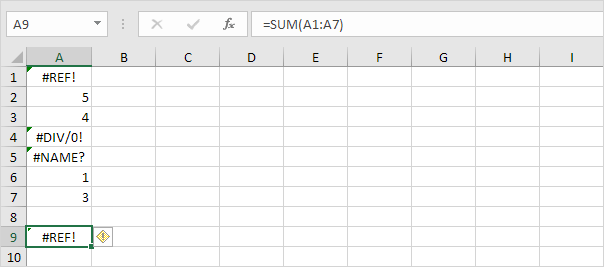2. Use the AGGREGATE function to sum a range with errors.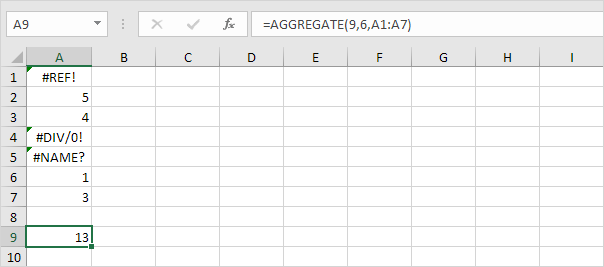Explanation: the first argument (9) tells Excel that you want to use the SUM function. The second argument (6) tells Excel that you want to ignore error values.

3. It's not easy to remember which argument belongs to which function. Fortunately, the AutoComplete feature in Excel helps you with this.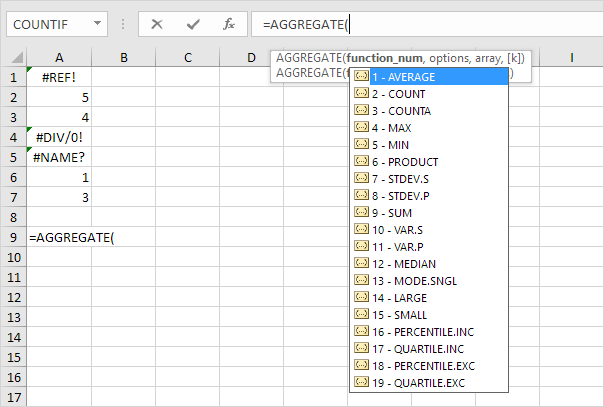4. The AGGREGATE function below finds the second largest number in a range with errors.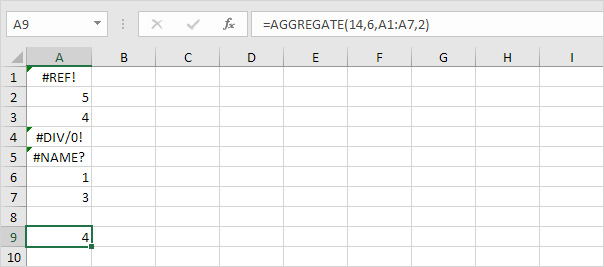Explanation: the first argument (14) tells Excel that you want to use the LARGE function. The second argument (6) tells Excel that you want to ignore error values. The fourth argument (2) tells Excel that you want to find the second largest number.

5. The AGGREGATE function below finds the maximum value ignoring error values and hidden rows.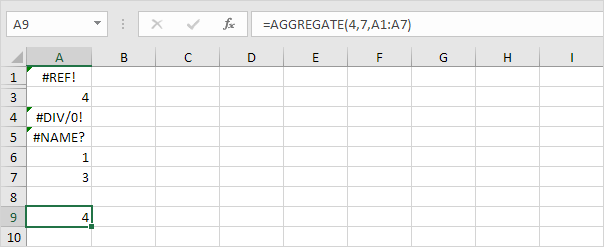Explanation: the first argument (4) tells Excel that you want to use the MAX function. The second argument (7) tells Excel that you want to ignore error values and hidden rows. In this example, row 2 is hidden.

Go to Next Chapter: Array Formulas